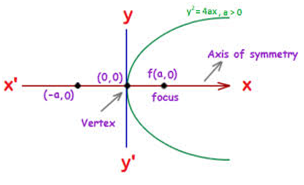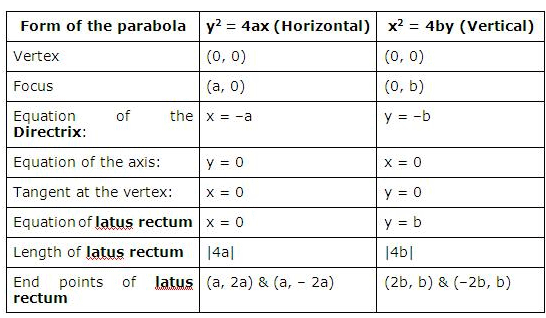Courses

# Notes | EduRev

## JEE: Notes | EduRev

The document Notes | EduRev is a part of the JEE Course Mock Test Series for JEE Main & Advanced 2022.
All you need of JEE at this link: JEE
• The general equation of a conic is ax2 + 2hxy + by2 + 2gx + 2fy +c =0. Here if e =1 and D≠ 0, then it represents a parabola.
• The general equation of parabola is (y-y0)= (x-x0), which has its vertex at (x0, y0).
• The general equation of parabola with vertex at (0, 0) is given by y2 = 4ax, and it opens rightwards.
• The parabola x2 = 4ay opens upwards.
• The equation y2 = 4ax is considered to be the standard equation of the parabola for which the various components are1. Vertex at (0,0)
2. Directrix is x+a = 0
3. Axis is y = 0
4. Focus is (a, 0)
5. Length of latus rectum = 4a
6. Ends of latus rectum are L(a, 2a) and L’(a, -2a)
• The parabola y = a(x – h)2 + khas its vertex at (h, k)
• The perpendicular distance from focus on directrix is half the length of latus rectum
• Vertex is the middle point of the focus and the point of intersection of directrix and axis
• Two parabolas are said to be equal if they have the same latus rectum
• The point (x1, y1) lies outside, on or inside the parabola y2 = 4ax, according as the expression y12 = 4ax1 is positive, zero or negative.
• Length of the chord intercepted by the parabola on the line y = mx + c is (4/m2) √a(1+m2) (a-mc)
• Length of the focal chord which makes an angle δ with the x-axis is 4a cosec2δ
• In parametric form, the parabola is represented by the equations x = at2 and y =2at
• The equation of a chord joining t1 and t2 is given by 2x – (t1 + t2) y + 2at1t2 = 0
• If a chord joining t1, t2 and t3, t4 pass through a point (c, 0) on the axis, then t1t2 = t3t4 = -c/a
• Tangents to the parabola y2 = 4ax
1. yy1 = 2a(x+x1) at the point (x1, y1)
2. y = mx + a/m ( m ≠ 0) at (a/m2, 2a/m)
3. ty = x+at2 at (at2, 2at)
• Normals to the parabola y2 = 4ax
1. y-y= -y1/ 2a(x-x1) at the point (x1, y1)
2. y = mx -2am – am3 at (am2, -2am)
3. y + tx = 2at +at3 at (at2, 2at)
• The equation of the director circle to the parabola is x + a = 0 which is same as the equation of the directrix
• The circle circumscribing the triangle formed by any three tangents to a parabola passes through the focus.
• The orthocenter of any triangle formed by three tangents to a parabola y2 = 4ax lies on then directrix and has the coordinates –a, a(t+ t2 + t3 + t1t2t3).
• The area of the triangle formed by three points on a parabola is twice the area of the triangle formed by the tangents at these points.
• A circle circumscribing the triangle formed by three co-normal points passes through the vertex of the parabola and its equation is given by 2(x2 + y2)– 2(h+2a)x - ky =0
The two vital parabolas along with their basic components like vertex and directrix are tabulated below:The document Notes | EduRev is a part of the JEE Course Mock Test Series for JEE Main & Advanced 2022.
All you need of JEE at this link: JEEUse Code STAYHOME200 and get INR 200 additional OFF Use Coupon Code
All Tests, Videos & Notes of JEE: JEE

### Up next### Top Courses for JEE## Mock Test Series for JEE Main & Advanced 2022

2 videos|258 docs|160 tests

### Up next### Top Courses for JEETrack your progress, build streaks, highlight & save important lessons and more!

,

,

,

,

,

,

,

,

,

,

,

,

,

,

,

,

,

,

,

,

,

;